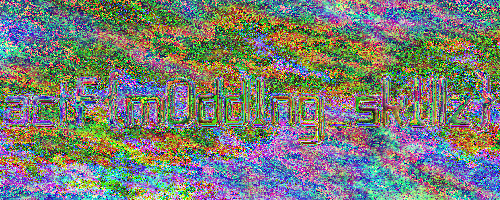Wacko Images [crypto]

# Wacko Images

How to make hiding stuff a e s t h e t i c? And can you make it normal again?

## Recon

We got an encrypted image and a source file how the image was created.

```...
key = [41, 37, 23]

a, b, c = img.shape

for x in range (0, a):
for y in range (0, b):
pixel = img[x, y]
for i in range(0,3):
pixel[i] = pixel[i] * key[i] % 251
img[x][y] = pixel
...
```

We see that every color of a pixel is multiplied by a key and the remainder when divided by 251 is saved as new value. Since we use a remainder value it is a bit of guess work what the original value should be.

In the end we ended up with this code, which gives us an image which shows some outlines of a flag.

## Code

```from numpy import *
from PIL import Image

flag = Image.open(r"enc.png")
img = array(flag)

key = [41, 37, 23]

a, b, c = img.shape

for x in range (0, a):
for y in range (0, b):
pixel = img[x, y]
for i in range(0,3):
pixel[i] = (251* pixel[i] + pixel[i]) / key[i]
img[x][y] = pixel

enc = Image.fromarray(img)
enc.save('flag.png')
```## Flag

`actf{m0dd1ng_sk1llz}`